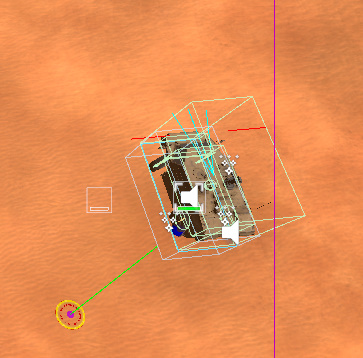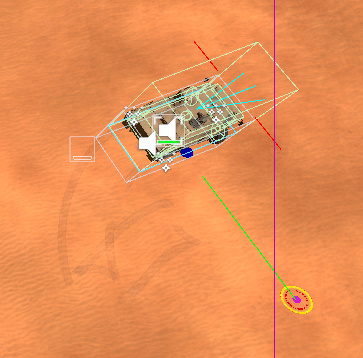# NavmeshCut, strange start points

#1

My units got strange starting points, because the NavmeshCut rectange has different side lengths. Most of the vehicles ar longer then wider, for them I got a start point for the path right side or left side, because that is the closest point to the walkable area. The cutted mesh part under the vehicle is not a walkable area. The following code calculates a point on the NavmeshCut rectange based on the target position.

Advantage: In most cases this gives you a better start point.

Disadvantage: If there is a non walkable area between target and vehicle, then the start point may not always the best start point.

Is there any better solution to calculate the best start point around the NavmeshCut rectange?

``````protected Vector2 CenterOffset = new Vector2(2, 4);

void Avake() {
NavmeshCut nc = GetComponent<NavmeshCut>();
if (nc) {
// Just need the range to the side.
CenterOffset = new Vector2(nc.rectangleSize.x / 2, nc.rectangleSize.y/2);
}
}

public void SearchPath() {
// Get point on the NavmeshCut rect.
Vector3 dir = (this.TargetPosition - transform.position).normalized;
float angleDir = MetadescUtils.AngleDir(transform.forward, dir, Vector3.up);
float angle = Vector3.Angle(transform.forward, dir) * angleDir;
Vector3 dirRot = Quaternion.AngleAxis(angle, Vector3.up) * Vector3.forward;
Vector3 offset = new Vector3(dirRot.x * CenterOffset.x, dirRot.y, dirRot.z * CenterOffset.y);
Vector3 pos = transform.TransformPoint(offset);

seeker.StartPath(pos, this.TargetPosition, OnPathComplete, GRIDMASK);
}

// Utility function to get the angle direction.
static public float AngleDir(Vector3 fwd, Vector3 targetDir, Vector3 up) {
Vector3 perp = Vector3.Cross(fwd, targetDir);
float dir = Vector3.Dot(perp, up);

if (dir > 0.0f) {
return 1.0f;
} else if (dir < 0.0f) {
return -1.0f;
} else {
return 1.0f;
}
}
``````

#2

Hi

What do you mean by starting point in this case? Are you trying to create a unit that has a navmesh cut attached to it?
Or are these characters that are existing a vehicle? Or something else?

#3

Hi, I have a vehicle, which has a NavmeshCut component. If I want to move the vehicle a bit forward, I get a starting point on the side, because this is the closest point to the walkable area. But I want that the vehicle drives just forward. My first idea was to give an offset to the Vector.forward, so the starting point worked fine for forward movement. But that was not a good solution, moving backward didn’t worked anymore. So I decided for a more generic solution, that I described in the first comment.

The blue point is the modified starting point:#4

I guess, to free the Navmesh cut cutted mesh, so make it walkable and then get a path, would be an optimal solution. But in this case may too much units could lead to a preformance peek on the CPU. Not sure that freeing the NavmeshCut cutted graph would be processed at first and then the pathfinding. If there is a higher prio queue for freeing the NavmeshCut areas, then it would work. I can remember me that for grid the graph updates got a higher priority, which then delayed the pathfindig. NavmeshCut works much more faster, maybe freeing the NavmeshCut cutted area and then searching for path would work fine.

#5

Hi

You shouldn’t cut away ground below the agent itself. That will make it always stand on a point outside the navmesh.
If you need multiple types of units you can create two graphs of which only one uses navmesh cutting (use the latest beta to get that toggle in the graph inspector). Also take a look at this tutorial: https://arongranberg.com/astar/docs/multipleagenttypes.html